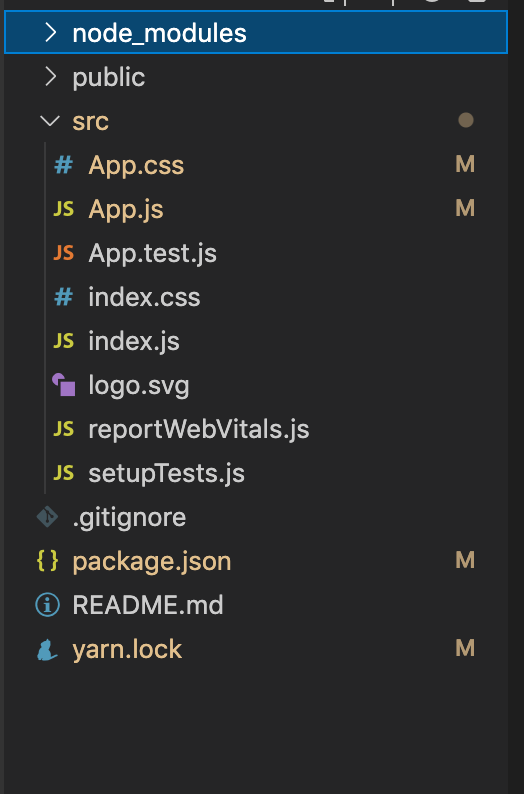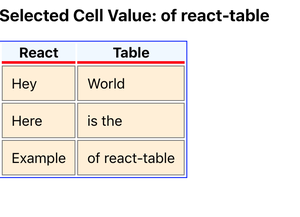# How to get cell value on React-Table ?

React-Table is a powerful library that allows you to create flexible and feature-rich tables in your React applications. It provides various functionalities to manipulate and interact with table data. One common requirement is to retrieve the value of a specific cell in React-Table. In this article, we will explore React Table.

Some Features of React Table:

• Lightweight (5kb-14kb+ depending on features used and tree-shaking).
• Auto out of the box, fully controllable API.
• Sorting (Multi and Stable)
• Filters.
• Pivoting & Aggregation.
• Virtualizable.
• Resizable

Get Cell Value: We can get the cell/column value of the table by adding the onClick event to the <td> tags.

`getCellValue function : onClick={()=> setCellValue(cell.value)}`

Creating React Application And Installing Module:

Step 1: Create a React application using the following command.

`npx create-react-app foldername`

Step 2: After creating your project folder i.e. foldername, move to it using the following command.

`cd foldername`

Step 3: After creating the ReactJS application, Install the react-table using the following command.

`npm i react-table`

Project Structure: It will look like the following:Project Structure

Example: Now write down the following code in the App.js file. Here, App is our default component where we have written our code.

## Javascript

 `import React, { useState } from ``'react'``;` `import { useTable } from ``'react-table'``;`   `const App = () => {`   `    ``// Define state` `    ``const [cellValue, setCellValue] = useState(``''``);`   `    ``// Add data here to show in table` `    ``const data = React.useMemo(` `        ``() => [` `            ``{` `                ``reactCol1: ``'Hey'``,` `                ``reactCol2: ``'World'``,` `            ``},` `            ``{` `                ``reactCol1: ``'Here'``,` `                ``reactCol2: ``'is the'``,` `            ``},` `            ``{` `                ``reactCol1: ``'Example'``,` `                ``reactCol2: ``'of react-table'``,` `            ``},` `        ``],` `        ``[]` `    ``)`   `    ``// Define column of the table` `    ``const columns = React.useMemo(` `        ``() => [` `            ``{` `                ``Header: ``'React'``,`   `                ``// Accessor is the "key" in the data` `                ``accessor: ``'reactCol1'``,` `            ``},` `            ``{` `                ``Header: ``'Table'``,` `                ``accessor: ``'reactCol2'``,` `            ``},` `        ``],` `        ``[]` `    ``)`   `    ``// Function to get cell value` `    ``const getCellValue = (cell) => {` `        ``setCellValue(cell.value)` `    ``}`   `    ``// Create the instance of table by ` `    ``// using hooks of react-table` `    ``const {` `        ``getTableProps,` `        ``getTableBodyProps,` `        ``headerGroups,` `        ``rows,` `        ``prepareRow,` `    ``} = useTable({ columns, data })`   `    ``return` `(` `        ``<>` `            ``{``/* Showing cell value */``}` `            ``

Selected Cell Value: {cellValue}

` `            ```   `                ``
` `                    ``{headerGroups.map(headerGroup => (` `                        ``` `                            ``{headerGroup.headers.map(column => (` `                                ``` `                                    ``{column.render(``'Header'``)}` `                                ``` `                            ``))}` `                        ``` `                    ``))}` `                ``` `                ``
` `                    ``{rows.map(row => {` `                        ``prepareRow(row)` `                        ``return` `(` `                            ``` `                                ``{row.cells.map(cell => {` `                                    ``return` `(` `                                        ``<>` `                                            ``{``/* Here added onClick function ` `                                            ``to get cell value */``}` `                                            `` getCellValue(cell)}` `                                                ``{...cell.getCellProps()}` `                                                ``style={{` `                                                    ``padding: ``'10px'``,` `                                                    ``border: ``'solid 1px gray'``,` `                                                    ``background: ``'papayawhip'``,` `                                                ``}}` `                                            ``>` `                                                ``{cell.render(``'Cell'``)}` `                                            ``` `                                        ``` `                                    ``)` `                                ``})}` `                            ``` `                        ``)` `                    ``})}` `                ``` `            ``` `        ``` `    ``)` `}`   `export ``default` `App;`

Step to Run Application: Run the application using the following command from the root directory of the project.

`npm start`

Output: Now open your browser and go to http://localhost:3000/, you will see the following output by clicking the of react-table cell.Output

Whether you're preparing for your first job interview or aiming to upskill in this ever-evolving tech landscape, GeeksforGeeks Courses are your key to success. We provide top-quality content at affordable prices, all geared towards accelerating your growth in a time-bound manner. Join the millions we've already empowered, and we're here to do the same for you. Don't miss out - check it out now!

Previous
Next